## Plus Blog

December 20, 2013

The central idea of applied statistics is that you can say something about a whole population by looking at a smaller sample. Without this idea there wouldn't be opinion polls, the social sciences would be stuffed, and there would be no way of testing new medical drugs, or the safety of bridges, etc, etc. It's the central limit theorem that is to a large extent responsible for the fact that we can do all these things and get a grip on the uncertainties involved.

Suppose that you want to know the average weight of the population in the UK. You go out and measure the weight of, say, 100 people whom you've randomly chosen and work out the average for this group — call this the sample average. Now the sample average is supposed to give you a good idea of the nation's average. But what if you happened to pick only fat people for your sample, or only very skinny ones?

To get an idea of how representative your average is likely to be, you need to know something about how the average weight of 100-people-samples varies over the population: if you took lots and lots of samples of size 100 and worked out the average weight for each, then how variable would this set of numbers be? And what would its average (the average of averages) be compared to the true average weight in the population?

For example, suppose you know that if you took lots and lots of 100- people-samples and wrote down the average weight of each sample, you'd get all values from 10kg to 300kg in equal proportion. Then this would tell you that your method of estimating the overall average by taking one sample of a 100 people isn't a very good one, because there's too much variability — you're just as likely to get any of the possible values, and you don't know which one is closest to the true average weight in the population.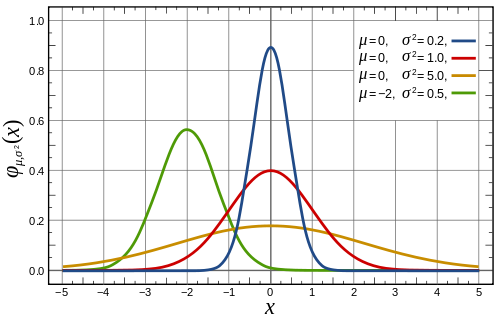Four versions of the normal distribution with different means and variances.

So how can we say anything about the distribution of 100-people-averages — called the sampling distribution — when we don't know anything about the distribution of weight across the population? This is where the central limit theorem comes in: it says that for a big enough sample (usually sampling 30 people is good enough) your sampling distribution is approximated by a normal distribution — this is the distribution with the famous bell shape.

The mean of this normal distribution (the average of averages corresponding to the tip of the bell) is the same as the mean in the population (the average weight of the population). The variance of this normal distribution, that is how much it varies about the mean (indicated by the width of the bell), depends on the sample size: the larger the sample, the smaller the variance. There's an equation which gives the exact relationship.

So if your sample size is big enough (100 would certainly do since it's bigger than 30), then the relatively small variance of the normal sampling distribution means that the average weight you observe is close to the mean of that normal distribution (since the bell is quite narrow). And since the mean of that normal distribution is equal to the true average weight across the population, your observed average is a good approximation of the true average.

You can make all this precise, for example you can say exactly how confident you are that the true average is within a certain distance of your sample average, and you can also use the result to calculate how large a sample you need to get an estimate of a given accuracy. It's the central limit theorem that lends precision to the art of statistical inference, and it's also behind the fact that the normal distribution is so ubiquitous.

The central limit theorem is actually a bit more general than we've let on here. See here for a precise statement.

Return to the Plus Advent Calendar

No comments yet
December 19, 2013

The dramatic curved surfaces of some of the iconic buildings created in the last decade, such as 30 St Mary's Axe (AKA the Gherkin) in London, are only logistically and economically possible thanks to mathematics. Curved panels of glass or other material are expensive to manufacture and to fit. Surprisingly, the curved surface of the Gherkin has been created almost entirely out of flat panels of glass — the only curved piece is the cap on the very top of the building. And simple geometry is all that is required to understand how.A geodesic sphere.

One way of approximating a curved surface using flat panels is using the concept of geodesic domes and surfaces. A geodesic is just a line between two points that follows the shortest possible distance — on the Earth the geodesic lines are great circles, such as the lines of longitude or the routes aircraft use for long distances. A geodesic dome is created from a lattice of geodesics that intersect to cover the curved surface with triangles. The mathematician Buckminster Fuller perfected the mathematical ideas behind geodesic domes and hoped that their properties — greater strength and space for minimum weight — might be the future of housing.

To try to build a sphere out of flat panels, such as a geodesic sphere, you first need to imagine an icosahedron (a polyhedron made up of 20 faces that are equilateral triangles) sitting just inside your sphere, so that the points of the icosahedron just touch the sphere's surface. An icosahedron, with its relatively large flat sides, isn't going to fool anyone into thinking it's curved. You need to use smaller flat panels and a lot more of them.

Divide each edge of the icosahedron in half, and join the points, dividing each of the icosahedron's faces into four smaller triangles. Projecting the vertices of these triangles onto the sphere (pushing them out a little til they two just touch the sphere's surface) now gives you a polyhedron with 80 triangular faces (which are no longer equilateral triangles) that gives a much more convincing approximation of the curved surface of the sphere. You can carry on in this way, dividing the edges in half and creating more triangular faces, until the surface made up of flat triangles is as close to a curved surface as you would like.

Find out more about the Gherkin and other iconic buildings on Plus.

Return to the Plus Advent Calendar

1 comments
December 18, 2013

How would you go about adding up all the integers from 1 to 100? Tap them into a calculator? Write a little computer code? Or look up the general formula for summing integers?Carl Friedrich Gauss as depicted on the (now defunct) German 10 Marks note.

Legend has it that the task of summing those numbers was given to the young Carl Friedrich Gauss by his teacher at primary school, as a punishment for misbehaving. Gauss didn't have a calculator or computer, no one did at that time, but he came up with the correct answer within seconds. Here's how he did it.

Notice that you can sum the numbers in pairs, starting at either end. First you add 1 and 100 to get 101. Next it's 2 and 99, giving 101 again. The same for 3 and 98. Continuing like this, the last pair you get is 50 and 51 and they give 101 again. Altogether there are 50 pairs all adding to 101, so the answer is 50 x 101 = 5050. Easy — if you're Gauss.

Return to the Plus Advent Calendar

No comments yet
December 17, 2013

Sequences of numbers can have limits. For example, the sequence 1, 1/2, 1/3, 1/4, ... has the limit 0 and the sequence 0, 1/2, 2/3, 3/4, 4/5, ... has the limit 1.

But not all number sequences behave so nicely. For example, the sequence 1/2, 1/3, 2/3, 1/4, 3/4, 1/5, 4/5, ... keeps jumping up and down, rather than getting closer and closer to one particular number. We can, however, discern some sort of limiting behaviour as we move along the sequence: the numbers never become larger than 1 or smaller than 0. And what's more, moving far enough along the sequence, you can find numbers that get as close as you like to both 1 and 0. So both 0 and 1 have some right to be considered limits of the sequence — and indeed they are: 1 is the limit superior and 0 is the limit inferior, so-called for obvious reasons.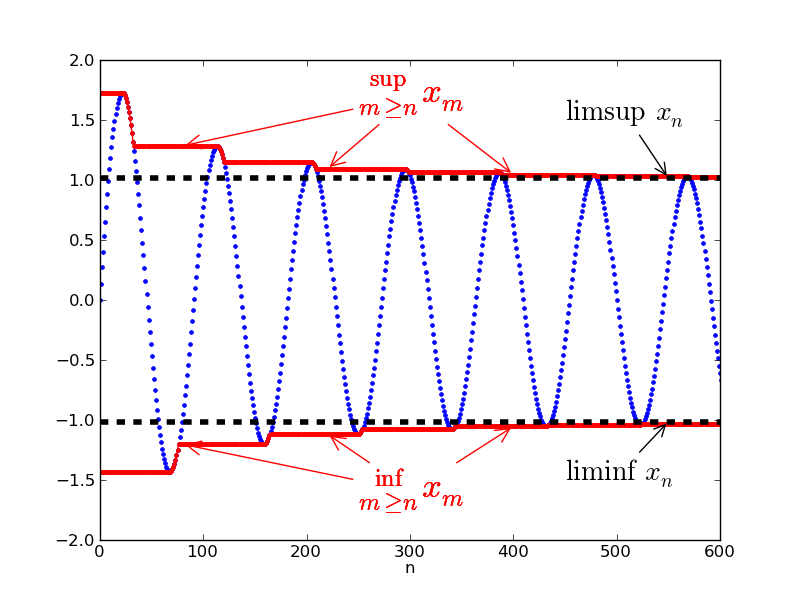But can you define these limits superior and inferior for a general sequence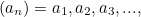for example the one shown in the picture? Here’s how to do it for the limit superior. First look at the whole sequence and find its least upper bound: that’s the smallest number that’s bigger than all the numbers in the sequence. Then chop off the first number in the sequence,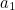, and again find the least upper bound for the new sequence. This might be smaller than the previous least upper bound (if that was equal to), but not bigger. Then chop off the first two numbers and again find the least upper bound.

Keep going, chopping off the first three, four, five, etc numbers, to get a sequence of least upper bounds (indicated by the red curve in the picture). In this sequence every number is either equal to or smaller than the number before. The limit superior is defined to be the limit of these least upper bounds. It always exists: since the sequence of least upper bounds is either constant or decreasing, it will either approach minus infinity or some other finite limit. The limit superior could also be equal to plus infinity, if there are numbers in the sequence that get arbitrarily large.

The limit inferior is defined in a similar way, only that you look at the sequence of greatest lower bounds and then take the limit of that.

You can read more about the limits inferior and superior in the Plus article The Abel Prize 2012.

Return to the Plus Advent Calendar

No comments yet
December 16, 2013

Here's a dilemma. Suppose you and a friend have been arrested for a crime and you're being interviewed separately. The police offer each of you the same deal. You can either confess, incriminating your partner, or remain silent. If you confess and your partner doesn't, then you get 2 years in jail (as a reward for talking), while your partner gets 10 years. If you both confess, then you both get 8 years (reduced from 10 years because at least you talked). If you both remain silent, you both get 5 years, as the evidence is only sufficient to convict you of a lesser crime.Solitary confinement

What should your strategy be? As a selfish and rational individual, you should talk. If your partner also talks, then your confession gets you 8 years instead of 10. If your partner doesn't talk, then it gets you 2 years instead of 5. Talking is your dominant strategy, it leaves you better off than silence, no matter what your partner does.

The trouble is that your partner, just as selfish and rational as you, will come to the same conclusion. You'll both decide to talk and get 8 years each. Paradoxically, your dominant strategy will leave both of you worse off than silence would have done.

The prisoner's dilemma is one of game theory's most famous games because it illustrates why people might refuse to cooperate when they would be better off doing so. One real-life situation that is similar to the dilemma is an arms race between two countries, in which both countries increase their military might when it would be better for both to disarm.

The dilemma has been used extensively in mathematical research into altruism. Mathematical research into altruism? Yes, that's right! Using the dilemma as the basis for computer simulations in which simulated individuals can either cooperate or defect has shown how altruism can evolve as a survival strategy, even in large societies.

To find out more, read Does it pay to be nice? And there's more about the prisoner's dilemma and economics in Adam Smith and the invisible hand.

Return to the Plus Advent Calendar

No comments yet
December 15, 2013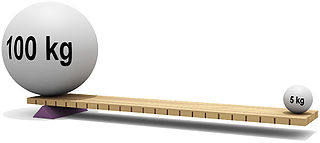Image © 2004 César Rincón.

Kneeling in the mud by a country road on a cold drizzly day recently, we finally appreciated the wonder that is a lever. We were trying to change a flat tyre and even jumping on the end of the wheel wrench wouldn't budge the wheel nuts. But when the AA arrived they undid them with ease, thanks to a wheel wrench that was three times the size of ours. There you have it ... size really does matter!

A lever is a truly remarkable device that can literally give any of us the strength of ten men. You can counteract 10 men pushing down on one side of a see-saw by applying just 1/10th of their force, as long as you are 10 times further from the see-saw's centre as they are. This is because the forces acting on a lever are proportional to the distances they are from the fulcrum. In this way a small amount of force moving a longer distance can move a large load over a smaller distance.

Levers are working hard all around us: in see-saws (where the fulcrum is between the loads), in wheel barrows (where the load is between the fulcrum and the force) and even in our very jaws (where the force is applied between the fulcrum and the load).

Archimedes was the first to mathematically describe how levers work and famously said: "Give me fulcrum and I shall move the Earth with a lever." And give us a long enough wheel wrench and we might just be able to change my next flat tyre for ourselves!

You can read more about mechanics on Plus.

Return to the Plus Advent Calendar

No comments yet